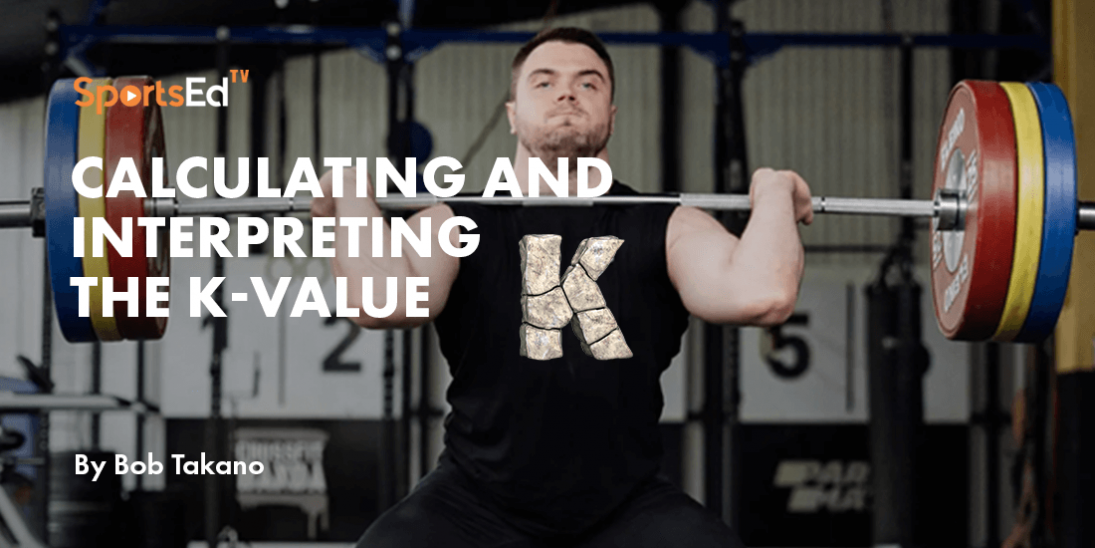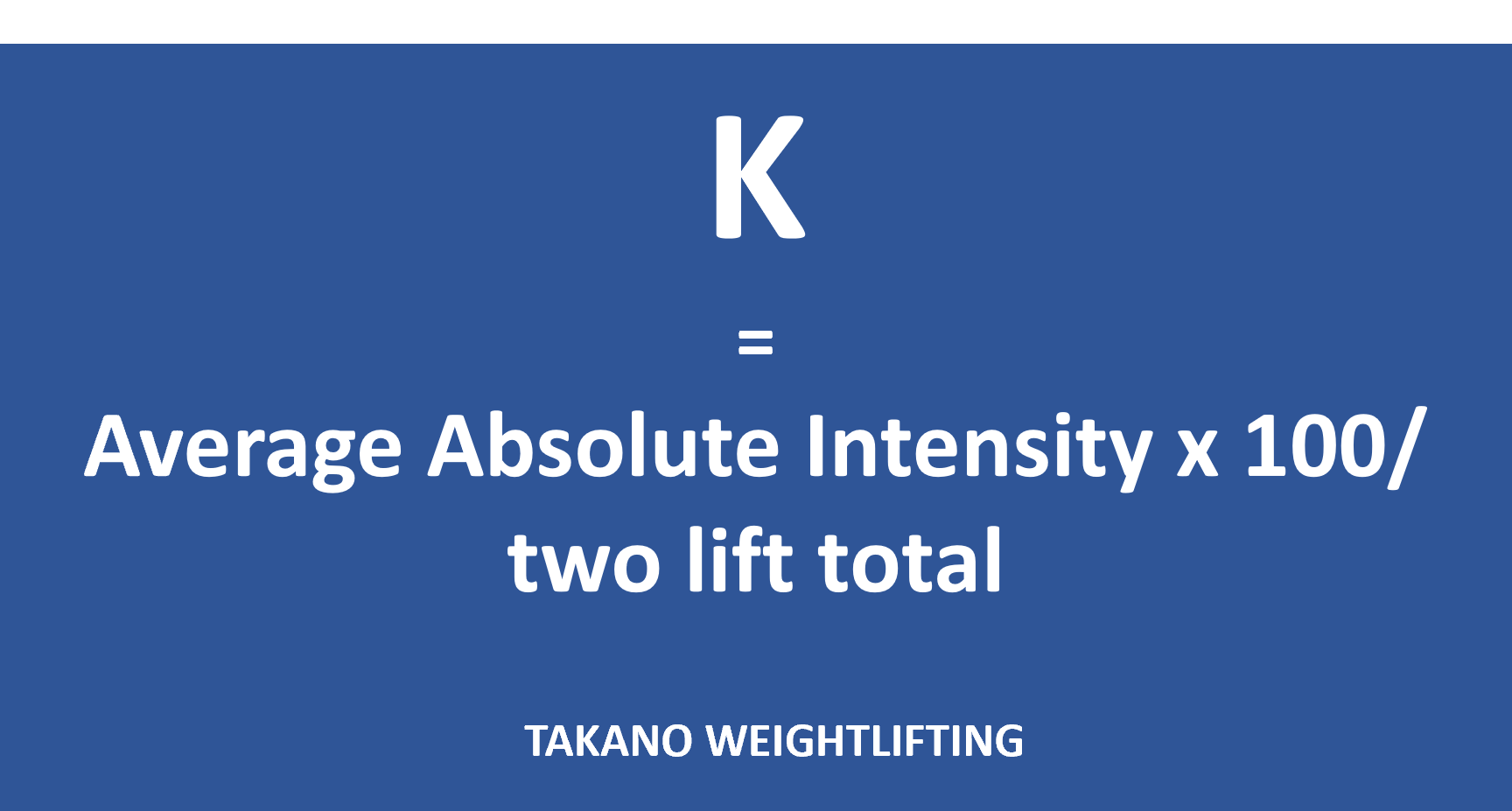# CALCULATING AND INTERPRETING THE K-VALUE IN WEIGHTLIFTING

Published: 2023-10-115/5 Average rating

In the previous two blog posts, I covered the processes of calculating the Average Relative Intensity (ARI) and the Average Absolute Intensity (AAI).  Today, I will cover the process for calculating the K-value and how to interpret it and modify programming.

First of all, there are three calculations of intensity.  The first two were covered in the previous two blogs: the average relative intensity and the average absolute intensity.  These two indicators enable the athlete and coach to determine whether or not the intensities are too heavy or too light.

The third indicator is called the K-value.  It is calculated by dividing the average absolute intensity by the two-lift total achieved at the conclusion of the macrocycle.  The exact formula is Average Absolute Intensity x 100/two lift total.  For most well-designed training programs, the figure will be in the range from 38 to 42.In short, calculate the Average Absolute Intensity for an entire macrocycle, multiply it by 100, and then divide it by the total achieved at the end of the competition mesocycle.

When the athlete has an exceptional meet at the end of the macrocycle, meaning significant PRs in both snatch and clean & jerk, the K-value for that macrocycle is the optimal one for that athlete, and the goal moving forward would be to attempt to achieve that K-value for each macrocycle.  A mature athlete will probably take several macrocycles to determine that ideal K-value.

If the clean & jerk achieved at the end of the macrocycle is significantly higher than 125% of the snatch, then the K-value should be lowered.  If the snatch result is significantly higher than 82% of the clean & jerk, the K-value should be raised moving forward.

While the ARI and AAI are both good intensity indicators, the K-value relates intensity to the competition total and, thus, is a better tool for adjusting the intensity.

These calculations represent a lot of record-keeping and arithmetic, but they allow the coach to more thoroughly nurture the process of athlete development.

Understanding Derived Indicators of Training Intensity

Figuring Out the Average Relative Intensity